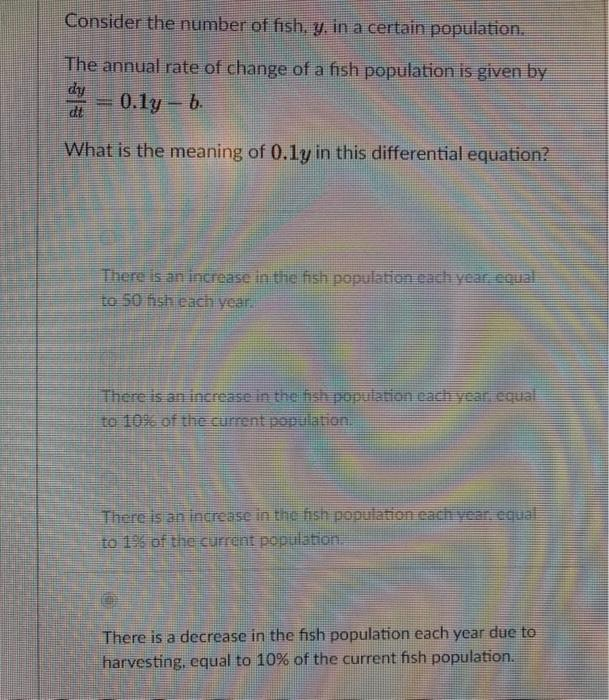# Question Consider the number of fish, y, in a certain population. The annual rate of change of a fish population is given by dy 0.1g - 6. What is the meaning of 0.ly in this differential equation? There is an increase in the fish population each year equal to 50 hsh each year There is an increase in the population cach year equal to 10% of the current population, There is an increase in the fish population each year, equal to 196 of the current population There is a decrease in the fish population each year due to harvesting, equal to 10% of the current fish population.